# Use examples¶

This jupyter notebook can be found at: https://github.com/environmentalscience/essm/blob/master/docs/examples/api_features.ipynb

Below, we will import some generic python packages that are used in this notebook:

:

# Checking for essm version installed
import pkg_resources
pkg_resources.get_distribution("essm").version

:

'0.4.3.dev21+dirty'

:

from IPython.core.display import display, HTML
display(HTML("<style>.container { width:120% !important; }</style>"))

:

from IPython.display import display
from sympy import init_printing, latex
init_printing()
from sympy.printing import StrPrinter
StrPrinter._print_Quantity = lambda self, expr: str(expr.abbrev)    # displays short units (m instead of meter)

:

import scipy as sc
# Import various functions from sympy
from sympy import Derivative, Eq, exp, log, solve, Symbol
from essm.equations import Equation
from essm.variables import Variable
from essm.variables.utils import ListTable, generate_metadata_table


## Importing variables and equations from essm¶

:

from essm.variables.chamber import *
from essm.variables.leaf import *
from essm.variables.physics.thermodynamics import *
from essm.equations.leaf import *
from essm.equations.physics.thermodynamics import *


## Plotting¶

Below, we will define a function to make plotting of equations very easy, and then show an example:

:

import matplotlib.pyplot as plt
from sympy import latex
from sympy import N
from numpy import arange
from essm.variables.units import derive_unit, SI, Quantity
from essm.variables.utils import markdown

def plot_expr2(xvar_min_max, yldata, yllabel=None, yrdata=None,
yrlabel='', clf=True, npoints=100, ylmin=None, ylmax=None,
yrmin=None, yrmax=None, xlabel=None,
colors=None,
loc_legend_left='best', loc_legend_right='right',
linestylesl=['-', '--', '-.', ':'],
linestylesr=['-', '--', '-.', ':'],
fontsize=None, fontsize_ticks=None, fontsize_labels=None,
fontsize_legend=None,
fig1=None, **args):
'''
Plot expressions as function of xvar from xmin to xmax.

**Examples:**

from essm.variables import Variable
from essm.variables.physics.thermodynamics import T_a
from essm.equations.physics.thermodynamics import eq_nua, eq_ka
vdict = Variable.__defaults__.copy()
expr = eq_nua.subs(vdict)
exprr = eq_ka.subs(vdict)
xvar = T_a
yldata = [(expr.rhs, 'full'), (expr.rhs/2, 'half')]
yrdata = exprr
plot_expr2((T_a, 273, 373), yldata, yllabel = (nu_a), yrdata=yrdata)
plot_expr2((T_a, 273, 373), yldata, yllabel = (nu_a),
yrdata=[(1/exprr.lhs, 1/exprr.rhs)],
loc_legend_right='lower right')
plot_expr2((T_a, 273, 373), expr)
plot_expr2((T_a, 273, 373), yldata, yllabel = (nu_a))
'''
(xvar, xmin, xmax) = xvar_min_max
if not colors:
if yrdata is not None:
colors = ['black', 'blue', 'red', 'green']
else:
colors = ['blue', 'black', 'red', 'green']
if fontsize:
fontsize_labels = fontsize
fontsize_legend = fontsize
fontsize_ticks = fontsize
if not fig1:
plt.close
plt.clf
fig = plt.figure(**args)
else:
fig = fig1
if hasattr(xvar, 'definition'):
unit1 = derive_unit(xvar)
if unit1 != 1:
strunit = ' (' + markdown(unit1) + ')'
else:
strunit = ''
if not xlabel:
xlabel = '$'+latex(xvar)+'$'+ strunit
else:
if not xlabel:
xlabel = xvar
if hasattr(yldata, 'lhs'):
yldata = (yldata.rhs, yldata.lhs)
if not yllabel:
if type(yldata) is tuple:
yllabel = yldata
else:
try:
yllabel = yldata
except Exception as e1:
print(e1)
print('yldata must be equation or list of (expr, name) tuples')

if type(yllabel) is not str:
unit1 = derive_unit(yllabel)
if unit1 != 1:
strunit = ' (' + markdown(unit1) + ')'
else:
strunit = ''

yllabel = '$'+latex(yllabel)+'$'+ strunit
if type (yldata) is not list and type(yldata) is not tuple:
# If only an expression given
yldata = [(yldata, '')]
if type(yldata) is not tuple:
yldata = [yldata]
if yrdata is not None:
if yrlabel == '':
if hasattr(yrdata, 'lhs'):
yrlabel = yrdata.lhs
if type (yrdata) is not list and type(yrdata) is not tuple:
# If only an expression given
yrdata = [yrdata]
if type(yrlabel) is not str:
yrlabel = '$'+latex(yrlabel)+'$'+ ' (' + markdown(derive_unit(yrlabel)) + ')'

xstep = (xmax - xmin)/npoints
xvals = arange(xmin, xmax, xstep)

ax1 =  fig.add_subplot(1, 1, 1)
if yrdata is not None:
color = colors
else:
color = 'black'
if ylmin:    ax1.set_ylim(ymin=float(ylmin))
if ylmax:    ax1.set_ylim(ymax=float(ylmax))
ax1.set_xlabel(xlabel)
ax1.set_ylabel(yllabel, color=color)
ax1.tick_params(axis='y', labelcolor=color)
i = 0
for (expr1, y1var) in yldata:
linestyle = linestylesl[i]
if yrdata is None:
color = colors[i]
i= i + 1
try:
y1vals = [expr1.subs(xvar, dummy).n() for dummy in xvals]
ax1.plot(xvals, y1vals, color=color, linestyle=linestyle, label=y1var)
except Exception as e1:
print([expr1.subs(xvar, dummy) for dummy in xvals])
print(e1)
if i > 1 or yrdata is not None:
plt.legend(loc=loc_legend_left, fontsize=fontsize_legend)

if yrdata is not None:
ax2 = ax1.twinx()  # instantiate a second axes that shares the same x-axis
color = colors
ax2.set_ylabel(yrlabel, color=color)
i = 0

for item in yrdata:
if type(item) is tuple:   # if item is tuple
(expr2, y2var) = item
else:
try:
(y2var, expr2) = (item.lhs, item.rhs)
except Exception as e1:
print(e1)
print('yrdata must be a list of equations or tuples (var, expr)')
return
linestyle = linestylesr[i]
i = i + 1
try:
y2vals = [expr2.subs(xvar, dummy).n() for dummy in xvals]
ax2.plot(xvals, y2vals, color=color, linestyle=linestyle, label=y2var)
except Exception as e1:
print(expr2)
print([expr2.subs(xvar, dummy).n() for dummy in xvals])
print(e1)

if not yrlabel:
if hasattr(yrdata, 'lhs'):
yrlabel = yrdata.lhs

if type(yrlabel) is not str:
yrlabel = '$'+latex(yrlabel)+'$'+ ' (' + markdown(derive_unit(yrlabel)) + ')'
ax2.tick_params(axis='y', labelcolor=color)
if yrmin:    ax2.set_ylim(ymin=float(yrmin))
if yrmax:    ax2.set_ylim(ymax=float(yrmax))
leg=ax2.legend(loc=loc_legend_right, fontsize=fontsize_legend)
for item in ([ax2.xaxis.label, ax2.yaxis.label]):
item.set_fontsize(fontsize_labels)
ax2.tick_params(axis='both', which='major', labelsize=fontsize_ticks)

for item in ([ax1.xaxis.label, ax1.yaxis.label]):
item.set_fontsize(fontsize_labels)
ax1.tick_params(axis='both', which='major', labelsize=fontsize_ticks)
fig.tight_layout()  # otherwise the right y-label is slightly clipped
return fig

:

vdict = Variable.__defaults__.copy()
expr = eq_nua.subs(vdict)
exprr = eq_ka.subs(vdict)
xvar = T_a
yldata = [(expr.rhs, 'full'), (expr.rhs/2, 'half')]
yrdata = exprr
fig=plot_expr2((T_a, 273, 373), yldata=expr, yrdata=exprr, yrmin=-0.0001, fontsize=14) # note that yrmin=0 would have no effect
fig=plot_expr2((T_a, 273, 373), yldata=expr, yrdata=exprr, colors=['red', 'blue'], linestylesr=['--'])
fig=plot_expr2((T_a, 273, 373), yldata, yllabel = (nu_a), yrdata=yrdata)
fig=plot_expr2((T_a, 273, 373), yldata, yllabel = (nu_a), yrdata=[(1/exprr.rhs, 1/exprr.lhs)],
loc_legend_right='lower right')
fig=plot_expr2((T_a, 273, 373), expr)
fig=plot_expr2((T_a, 273, 373), yldata, yllabel = (nu_a))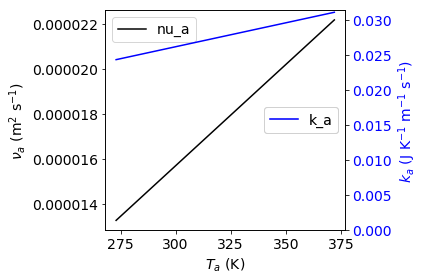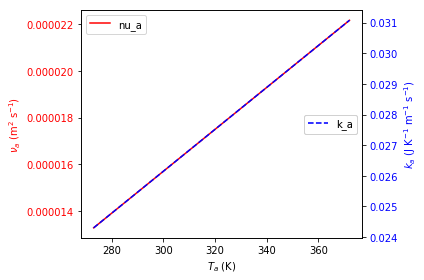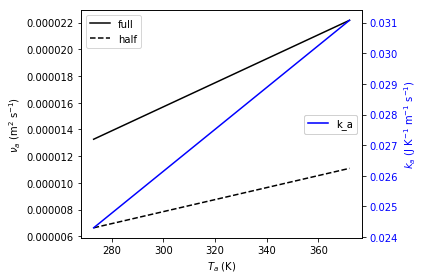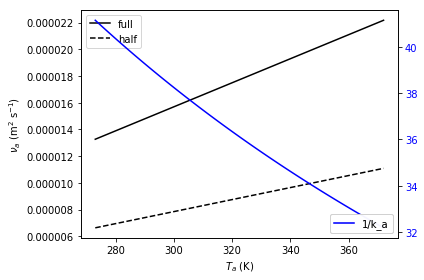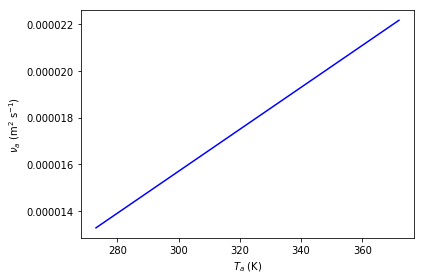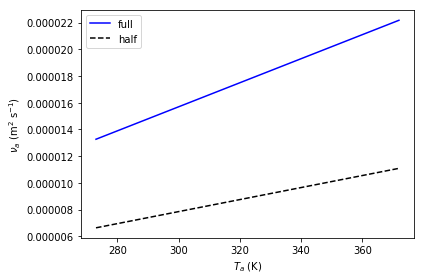We can also manipulate the figure later, e.g. change the width:

:

%matplotlib inline
fig.set_figwidth(8)
fig

:## Creating new variables¶

To create custom variables, first import Variable:

:

from essm.variables import Variable


To define units, you can either import these units from the library, e.g.

from essm.variables.units import joule, kelvin, meter

or import the appropriate units from sympy, e.g.

from sympy.physics.units import joule, kelvin, meter

:

from sympy.physics.units import joule, kelvin, meter, mole, pascal, second


Then you can define a custom variable with its name, description, domain, latex_name, unit, and an optional default value, e.g.:

:

class R_mol(Variable):
"""Molar gas constant."""
unit = joule/(kelvin*mole)
latex_name = 'R_{mol}'
default = 8.314472

/home/stan/Programs/essm/essm/variables/_core.py:89: UserWarning: "essm.variables.physics.thermodynamics:R_mol" will be overridden by "__main__:<class '__main__.R_mol'>"
instance[expr] = instance


The variables defined above hold information about their docstring, units, latex representations and default values if any. Each can be accessed by e.g.:

:

print(R_mol.__doc__)
print(R_mol.definition.unit)
print(R_mol.definition.latex_name)
print(R_mol.definition.default)

Molar gas constant.
J/(K*mol)
R_{mol}
8.314472


We will now define a few additional variables.

:

class P_g(Variable):
"""Pressure of gas."""
unit = pascal

class V_g(Variable):
"""Volume of gas."""
unit = meter**3

class n_g(Variable):
"""Amount of gas."""
unit = mole

class n_w(Variable):
"""Amount of water."""
unit = mole

class T_g(Variable):
"""Temperature of gas."""
unit = kelvin

class P_wa(Variable):
"""Partial pressure of water vapour in air."""
unit = pascal
latex_name = 'P_{wa}'

/home/stan/Programs/essm/essm/variables/_core.py:89: UserWarning: "essm.variables.physics.thermodynamics:P_wa" will be overridden by "__main__:<class '__main__.P_wa'>"
instance[expr] = instance


### Variables with expressions as definitions¶

:

class Delta_Pwa(Variable):
"""Slope of saturated vapour pressure, $\partial P_{wa} / \partial T_g$"""
expr = Derivative(P_wa,T_g)
latex_name = r'\Delta'

:

Delta_Pwa.definition.unit

:

$$\frac{Pa}{K}$$
:

Delta_Pwa.definition.expr

:

$$\frac{d}{d T_g} P_{wa}$$
:

generate_metadata_table([Delta_Pwa])

:

 Symbol Name Description Definition Default value Units $\Delta$ Delta_Pwa Slope of saturated vapour pressure, $\partial P_{wa} / \partial T_g$ $\frac{d}{d T_g} P_{wa}$ - Pa K$^{-1}$

### Linking assumptions to variables¶

We can specify if a given variable is a complex, real, integer etc. by using the assumptions property during variable definition:

:

class x(Variable):
"""Positive real variable."""
assumptions = {'positive': True, 'real': True}

print(solve(x**2 - 1))




## Creating new equations¶

Equations have a left hand side and a right hand side and if they contain variables with units, the units of each addend must be the same.

### Custom equation¶

To create custom equations, first import Equation:

:

from essm.equations import Equation


We will now define an equation representing the ideal gas law, based on the variables defined above:

:

class eq_ideal_gas_law(Equation):
"""Ideal gas law."""

expr = Eq(P_g*V_g, n_g*R_mol*T_g)


Note that whenever an equation is defined, its units are checked for consistency in the background and if they are not consistent, an error message will be printed. To illustrate this, we will try to define the above equation again, but omit temperature on the right hand side:

:

try:
class eq_ideal_gas_law(Equation):
"""Ideal gas law."""

expr = Eq(P_g*V_g, n_g*R_mol)
except Exception as exc1:
print(exc1)

Dimension of "R_mol*n_g" is Dimension(length**2*mass/(temperature*time**2)), but it should be the same as P_g*V_g, i.e. Dimension(length**2*mass/time**2)


The equation can be displayed in typesetted form, and the documentation string can be accessed in a similar way as for Variable:

:

display(eq_ideal_gas_law)
print(eq_ideal_gas_law.__doc__)

$$P_g V_g = R_{mol} T_g n_g$$
Ideal gas law.


### New equation based on manipulation of previous equations¶

We can use the above equation just as any Sympy expression, and e.g. solve it for pressure:

:

soln = solve(eq_ideal_gas_law, P_g, dict=True); print(soln)

[{P_g: R_mol*T_g*n_g/V_g}]


If we want to define a new equation based on a manipulation of eq_ideal_gas_law we can specify that the parent of the new equation is eq_ideal_gas_law.definition:

:

class eq_Pg(eq_ideal_gas_law.definition):
"""Calculate pressure of ideal gas."""

expr = Eq(P_g, soln[P_g])
eq_Pg

:

$$P_g = \frac{R_{mol} T_g n_g}{V_g}$$

We can also have nested inheritance, if we now define another equation based on eq_Pg:

:

class eq_Pwa_nw(eq_Pg.definition):
"""Calculate vapour pressure from amount of water in gas."""

expr = Eq(P_wa, eq_Pg.rhs.subs(n_g, n_w))
eq_Pwa_nw

:

$$P_{wa} = \frac{R_{mol} T_g n_w}{V_g}$$

### Show inheritance of equations¶

To see the inheritance (what other equations it depends on) of the newly created equation:

:

eq_Pwa_nw.definition.__bases__

:

(__main__.eq_Pg,)

:

[parent.name for parent in eq_Pwa_nw.definition.__bases__]

:

['eq_Pg']

:

[parent.expr for parent in eq_Pwa_nw.definition.__bases__]

:

$$\left [ P_g = \frac{R_{mol} T_g n_g}{V_g}\right ]$$

We can also use a pre-defined function to extract the set of parents:

:

from essm.variables.utils import get_parents
get_parents(eq_Pwa_nw)

:

{'eq_Pg'}


We can use another function to get all parents recursively:

:

from essm.variables.utils import get_allparents
get_allparents(eq_Pwa_nw)

:

{'eq_Pg', 'eq_ideal_gas_law'}


### Computational burden of deriving equations within class definition¶

If we solve for a variable to derive a new equation, is the solve() command performed every time this equation is used?

:

class eq_Pg1(eq_ideal_gas_law.definition):
"""Calculate pressure of ideal gas."""
from sympy import solve
soln = solve(eq_ideal_gas_law, P_g, dict=True); print(soln)
expr = Eq(P_g, soln[P_g])
eq_Pg1

[{P_g: R_mol*T_g*n_g/V_g}]

/home/stan/Programs/essm/essm/equations/_core.py:107: UserWarning: "__main__:eq_Pg" will be overridden by "__main__:<class '__main__.eq_Pg1'>"
instance[expr] = instance

:

$$P_g = \frac{R_{mol} T_g n_g}{V_g}$$
:

%time
eq_Pg.subs({R_mol: 8.314, T_g: 300, n_g: 0.1, V_g: 1})

CPU times: user 3 µs, sys: 2 µs, total: 5 µs
Wall time: 8.34 µs

:

$$P_g = 249.42$$
:

%time
eq_Pg1.subs({R_mol: 8.314, T_g: 300, n_g: 0.1, V_g: 1})

CPU times: user 3 µs, sys: 1 µs, total: 4 µs
Wall time: 7.87 µs

:

$$P_g = 249.42$$

There is actually no difference!

### Empirical equations with internal variables¶

Empirical equations not only contain variables but also numbers. As an example, we will try to define the Clausius-Clapeyron equation for saturation vapour pressure in the following example, after defining a few additional variables used in this equation.

$P_{wa} = 611 e^\frac{-M_w \lambda_E (1/T_g - 1/273)}{R_{mol}}$
:

from sympy.physics.units import joule, kilogram
class lambda_E(Variable):
"""Latent heat of evaporation."""
unit = joule/kilogram
latex_name = '\\lambda_E'
default = 2.45e6

class M_w(Variable):
"""Molar mass of water."""
unit = kilogram/mole
default = 0.018

/home/stan/Programs/essm/essm/variables/_core.py:89: UserWarning: "essm.variables.physics.thermodynamics:lambda_E" will be overridden by "__main__:<class '__main__.lambda_E'>"
instance[expr] = instance
/home/stan/Programs/essm/essm/variables/_core.py:89: UserWarning: "essm.variables.physics.thermodynamics:M_w" will be overridden by "__main__:<class '__main__.M_w'>"
instance[expr] = instance

:

from sympy import exp
try:
class eq_Pwa_CC(Equation):
"""Clausius-Clapeyron P_wa as function of T_g.

\cite[Eq. B3]{hartmann_global_1994}
"""

expr = Eq(P_wa, 611.*exp(-M_w*lambda_E*(1/T_g - 1/273.)/R_mol))
except Exception as exc1:
print(exc1)

Dimension of "1/T_g" is Dimension(1/temperature), but it should be the same as -0.00366300366300366, i.e. Dimension(1)


The unit mismatch reported in the error message stems from the fact that the numbers in the empirical equation actually need units. Since the term in the exponent has to be non-dimensional, the units of 611 must be the same as those of P_wa, i.e. pascal. The units of the subtraction term in the exponent must match, meaning that 273 needs units of kelvin. To avoid the error message, we can define the empirical numbers as internal variables to the equation we want to define:

:

class eq_Pwa_CC(Equation):
"""Clausius-Clapeyron P_wa as function of T_g.

Eq. B3 in :cite{hartmann_global_1994}
"""

class p_CC1(Variable):
"""Internal parameter of eq_Pwl."""
unit = pascal
latex_name = '611'
default = 611.

class p_CC2(Variable):
"""Internal parameter of eq_Pwl."""
unit = kelvin
latex_name = '273'
default = 273.

expr = Eq(P_wa, p_CC1*exp(-M_w*lambda_E*(1/T_g - 1/p_CC2)/R_mol))


In the above, we defined the latex representation of the empirical constants as their actual values, so the equation displays in the familiar way:

:

eq_Pwa_CC

:

$$P_{wa} = 611 e^{- \frac{M_w \lambda_E \left(- \frac{1}{273} + \frac{1}{T_g}\right)}{R_{mol}}}$$

All default values of variables defined along with the variable definitions are stored in a dictionary that can be accessed as Variable.__defaults__. We can substitute the values from this dictionary into our empirical equation to plot saturation vapour pressure as a function of temperature:

:

expr = eq_Pwa_CC.subs(Variable.__defaults__)
print(expr)
xvar = T_g
p = plot_expr2((xvar, 273, 373), expr)

Eq(P_wa, 167405731976.232*exp(-5304.00487246815/T_g))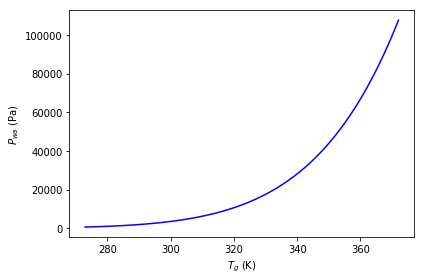### Piecewise defined equations¶

:

from sympy import Piecewise
expr = Eq(P_wa, Piecewise((0, T_a < 0), (eq_Pwa_CC.rhs, T_a >= 0)))
expr

:

$$P_{wa} = \begin{cases} 0 & \text{for}\: T_a < 0 \\611 e^{- \frac{M_w \lambda_E \left(- \frac{1}{273} + \frac{1}{T_g}\right)}{R_{mol}}} & \text{otherwise} \end{cases}$$
:

try:
class eq1(Equation):
"""Test"""
expr = Eq(P_wa, Piecewise((0, T_a < 0), (eq_Pwa_CC.rhs, T_a >= 0)))
display(eq1)
except Exception as e1:
print(e1)

$$P_{wa} = \begin{cases} 0 & \text{for}\: T_a < 0 \\611 e^{- \frac{M_w \lambda_E \left(- \frac{1}{273} + \frac{1}{T_g}\right)}{R_{mol}}} & \text{otherwise} \end{cases}$$

If the above returns a dimension error, then unit checking for Piecewise has not been implemented yet.

## Substituting into integrals and derivatives and evaluating¶

Above, we defined Delta_Pwa as a variable that represents the partial derivative of P_wa with respect to T_g:

class Delta_Pwa(Variable):
"""Slope of saturated vapour pressure, \$\partial P_{ws} / \partial T_g"""
expr = P_wa(T_g).diff(T_g)
#unit = pascal/kelvin
latex_name = r'\Delta'


This definition can be accessed by typing Delta_Pwa.definition.expr. Example:

:

print(Delta_Pwa.definition.expr)
display(Eq(Delta_Pwa, Delta_Pwa.definition.expr))

Derivative(P_wa, T_g)

$$\Delta = \frac{d}{d T_g} P_{wa}$$

We also defined the Clausius-Clapeyron approximation to $$P_{wa}(T_g)$$ as eq_Pwa_CC.

:

display(eq_Pwa_CC)
print(eq_Pwa_CC.__doc__)

$$P_{wa} = 611 e^{- \frac{M_w \lambda_E \left(- \frac{1}{273} + \frac{1}{T_g}\right)}{R_{mol}}}$$
Clausius-Clapeyron P_wa as function of T_g.

Eq. B3 in :cite{hartmann_global_1994}



If we want to substitute this approximation into Delta_Pwa.definition.expr, we need to use replace instead of subs and evaluate the derivative using doit():

:

expr = Eq(Delta_Pwa, Delta_Pwa.definition.expr.replace(P_wa, eq_Pwa_CC.rhs).doit())
display(expr)
p = plot_expr2((T_g, 273, 373), expr.subs(Variable.__defaults__))

$$\Delta = \frac{M_w \lambda_E 611 e^{- \frac{M_w \lambda_E \left(- \frac{1}{273} + \frac{1}{T_g}\right)}{R_{mol}}}}{R_{mol} T_g^{2}}$$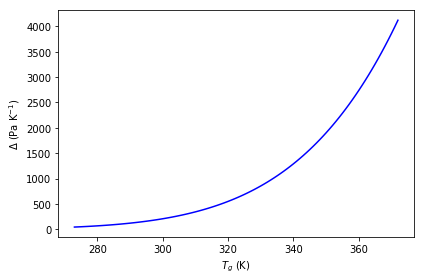If we only had the slope of the curve, we could take the integral to get the absolute value:

:

from sympy import Integral
class T_a1(Variable):
"""Air temperature"""
unit = kelvin
latex_name = r'T_{a1}'

class T_a2(Variable):
"""Air temperature"""
unit = kelvin
latex_name = r'T_{a2}'

class P_wa1(Variable):
"""P_wa at T1"""
unit = pascal
latex_name = r'P_{wa1}'

class eq_Pwa_Delta(Equation):
"""P_wa deduced from the integral of Delta"""
expr = Eq(P_wa, P_wa1 + Integral(Delta_Pwa, (T_g, T_a1, T_a2)))
display(eq_Pwa_Delta)

$$P_{wa} = P_{wa1} + \int_{T_{a1}}^{T_{a2}} \Delta\, dT_g$$
:

expr_Delta = eq_Pwa_CC.rhs.diff(T_g)
expr = Eq(P_wa, eq_Pwa_Delta.rhs.replace(Delta_Pwa, expr_Delta).doit())
vdict = Variable.__defaults__.copy()
vdict[T_a1] = 273.
vdict[P_wa1] = eq_Pwa_CC.rhs.subs(T_g, T_a1).subs(vdict)
display(expr.subs(vdict))
p = plot_expr2((T_a2, 273, 373), expr.subs(vdict))

$$P_{wa} = 167405731976.232 e^{- \frac{5304.00487246815}{T_{a2}}}$$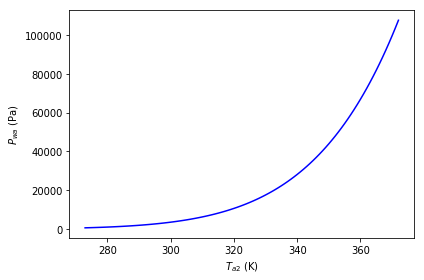## Unit conversions¶

Values for variables are often given in obscure units, but to convert to our standard units, we can use the dictionary SI_EXTENDED_DIMENSIONS:

:

from sympy.physics.units import convert_to, kilo, mega, joule, kilogram, meter, second, inch, hour
from essm.variables.units import SI_EXTENDED_DIMENSIONS, SI_EXTENDED_UNITS
value1 = 0.3
unit1 = inch/hour
print(value1*unit1)
unit2 = Variable.get_dimensional_expr(unit1).subs(SI_EXTENDED_DIMENSIONS)
print(convert_to(value1*unit1, unit2))

0.3*inch/hour
2.11666666666667e-6*m/s


## Exporting definitions¶

The below example creates a file called test_variable-definitions.py with all the variable definitions and relevant imports used in this notebook. Then, we re-import all the variables from the newly created file and create another file called test_equation-definitions.py with all the equation definitions and relevant imports. To re-use the equations in another notebook, just execute from test_equation-definitions.py import *

:

from essm._generator import EquationWriter, VariableWriter

:

StrPrinter._print_Quantity = lambda self, expr: str(expr.name)    # displays long units (meter instead of m)
writer = VariableWriter(docstring='Variables defined in api_features.ipynb and dependencies.')
for variable in Variable.__registry__.keys():
writer.var(variable)
writer.write('test_variable_definitions.py')
StrPrinter._print_Quantity = lambda self, expr: str(expr.abbrev)    # displays short units (m instead of meter)

:

from test_variable_definitions import *

/home/stan/Programs/essm/essm/variables/_core.py:89: UserWarning: "essm.variables.physics.thermodynamics:alpha_a" will be overridden by "test_variable_definitions:<class 'test_variable_definitions.alpha_a'>"
instance[expr] = instance
/home/stan/Programs/essm/essm/variables/_core.py:89: UserWarning: "essm.variables.physics.thermodynamics:c_pa" will be overridden by "test_variable_definitions:<class 'test_variable_definitions.c_pa'>"
instance[expr] = instance
/home/stan/Programs/essm/essm/variables/_core.py:89: UserWarning: "essm.variables.physics.thermodynamics:c_pamol" will be overridden by "test_variable_definitions:<class 'test_variable_definitions.c_pamol'>"
instance[expr] = instance
/home/stan/Programs/essm/essm/variables/_core.py:89: UserWarning: "essm.variables.physics.thermodynamics:c_pv" will be overridden by "test_variable_definitions:<class 'test_variable_definitions.c_pv'>"
instance[expr] = instance
/home/stan/Programs/essm/essm/variables/_core.py:89: UserWarning: "essm.variables.physics.thermodynamics:C_wa" will be overridden by "test_variable_definitions:<class 'test_variable_definitions.C_wa'>"
instance[expr] = instance
/home/stan/Programs/essm/essm/variables/_core.py:89: UserWarning: "essm.variables.physics.thermodynamics:D_va" will be overridden by "test_variable_definitions:<class 'test_variable_definitions.D_va'>"
instance[expr] = instance
/home/stan/Programs/essm/essm/variables/_core.py:89: UserWarning: "essm.variables.physics.thermodynamics:g" will be overridden by "test_variable_definitions:<class 'test_variable_definitions.g'>"
instance[expr] = instance
/home/stan/Programs/essm/essm/variables/_core.py:89: UserWarning: "essm.variables.physics.thermodynamics:Gr" will be overridden by "test_variable_definitions:<class 'test_variable_definitions.Gr'>"
instance[expr] = instance
/home/stan/Programs/essm/essm/variables/_core.py:89: UserWarning: "essm.variables.physics.thermodynamics:h_c" will be overridden by "test_variable_definitions:<class 'test_variable_definitions.h_c'>"
instance[expr] = instance
/home/stan/Programs/essm/essm/variables/_core.py:89: UserWarning: "essm.variables.physics.thermodynamics:k_a" will be overridden by "test_variable_definitions:<class 'test_variable_definitions.k_a'>"
instance[expr] = instance
/home/stan/Programs/essm/essm/variables/_core.py:89: UserWarning: "__main__:lambda_E" will be overridden by "test_variable_definitions:<class 'test_variable_definitions.lambda_E'>"
instance[expr] = instance
/home/stan/Programs/essm/essm/variables/_core.py:89: UserWarning: "essm.variables.physics.thermodynamics:Le" will be overridden by "test_variable_definitions:<class 'test_variable_definitions.Le'>"
instance[expr] = instance
/home/stan/Programs/essm/essm/variables/_core.py:89: UserWarning: "essm.variables.physics.thermodynamics:M_air" will be overridden by "test_variable_definitions:<class 'test_variable_definitions.M_air'>"
instance[expr] = instance
/home/stan/Programs/essm/essm/variables/_core.py:89: UserWarning: "essm.variables.physics.thermodynamics:M_N2" will be overridden by "test_variable_definitions:<class 'test_variable_definitions.M_N2'>"
instance[expr] = instance
/home/stan/Programs/essm/essm/variables/_core.py:89: UserWarning: "essm.variables.physics.thermodynamics:M_O2" will be overridden by "test_variable_definitions:<class 'test_variable_definitions.M_O2'>"
instance[expr] = instance
/home/stan/Programs/essm/essm/variables/_core.py:89: UserWarning: "__main__:M_w" will be overridden by "test_variable_definitions:<class 'test_variable_definitions.M_w'>"
instance[expr] = instance
/home/stan/Programs/essm/essm/variables/_core.py:89: UserWarning: "essm.variables.physics.thermodynamics:nu_a" will be overridden by "test_variable_definitions:<class 'test_variable_definitions.nu_a'>"
instance[expr] = instance
/home/stan/Programs/essm/essm/variables/_core.py:89: UserWarning: "essm.variables.physics.thermodynamics:Nu" will be overridden by "test_variable_definitions:<class 'test_variable_definitions.Nu'>"
instance[expr] = instance
/home/stan/Programs/essm/essm/variables/_core.py:89: UserWarning: "essm.variables.physics.thermodynamics:P_a" will be overridden by "test_variable_definitions:<class 'test_variable_definitions.P_a'>"
instance[expr] = instance
/home/stan/Programs/essm/essm/variables/_core.py:89: UserWarning: "essm.variables.physics.thermodynamics:Pr" will be overridden by "test_variable_definitions:<class 'test_variable_definitions.Pr'>"
instance[expr] = instance
/home/stan/Programs/essm/essm/variables/_core.py:89: UserWarning: "essm.variables.physics.thermodynamics:P_N2" will be overridden by "test_variable_definitions:<class 'test_variable_definitions.P_N2'>"
instance[expr] = instance
/home/stan/Programs/essm/essm/variables/_core.py:89: UserWarning: "essm.variables.physics.thermodynamics:P_O2" will be overridden by "test_variable_definitions:<class 'test_variable_definitions.P_O2'>"
instance[expr] = instance
/home/stan/Programs/essm/essm/variables/_core.py:89: UserWarning: "__main__:P_wa" will be overridden by "test_variable_definitions:<class 'test_variable_definitions.P_wa'>"
instance[expr] = instance
/home/stan/Programs/essm/essm/variables/_core.py:89: UserWarning: "essm.variables.physics.thermodynamics:P_was" will be overridden by "test_variable_definitions:<class 'test_variable_definitions.P_was'>"
instance[expr] = instance
/home/stan/Programs/essm/essm/variables/_core.py:89: UserWarning: "essm.variables.physics.thermodynamics:R_d" will be overridden by "test_variable_definitions:<class 'test_variable_definitions.R_d'>"
instance[expr] = instance
/home/stan/Programs/essm/essm/variables/_core.py:89: UserWarning: "essm.variables.physics.thermodynamics:Re_c" will be overridden by "test_variable_definitions:<class 'test_variable_definitions.Re_c'>"
instance[expr] = instance
/home/stan/Programs/essm/essm/variables/_core.py:89: UserWarning: "essm.variables.physics.thermodynamics:Re" will be overridden by "test_variable_definitions:<class 'test_variable_definitions.Re'>"
instance[expr] = instance
/home/stan/Programs/essm/essm/variables/_core.py:89: UserWarning: "essm.variables.physics.thermodynamics:rho_a" will be overridden by "test_variable_definitions:<class 'test_variable_definitions.rho_a'>"
instance[expr] = instance
/home/stan/Programs/essm/essm/variables/_core.py:89: UserWarning: "essm.variables.physics.thermodynamics:R_u" will be overridden by "test_variable_definitions:<class 'test_variable_definitions.R_u'>"
instance[expr] = instance
/home/stan/Programs/essm/essm/variables/_core.py:89: UserWarning: "__main__:R_mol" will be overridden by "test_variable_definitions:<class 'test_variable_definitions.R_mol'>"
instance[expr] = instance
/home/stan/Programs/essm/essm/variables/_core.py:89: UserWarning: "essm.variables.physics.thermodynamics:R_s" will be overridden by "test_variable_definitions:<class 'test_variable_definitions.R_s'>"
instance[expr] = instance
/home/stan/Programs/essm/essm/variables/_core.py:89: UserWarning: "essm.variables.physics.thermodynamics:sigm" will be overridden by "test_variable_definitions:<class 'test_variable_definitions.sigm'>"
instance[expr] = instance
/home/stan/Programs/essm/essm/variables/_core.py:89: UserWarning: "essm.variables.physics.thermodynamics:T0" will be overridden by "test_variable_definitions:<class 'test_variable_definitions.T0'>"
instance[expr] = instance
/home/stan/Programs/essm/essm/variables/_core.py:89: UserWarning: "essm.variables.physics.thermodynamics:T_a" will be overridden by "test_variable_definitions:<class 'test_variable_definitions.T_a'>"
instance[expr] = instance
/home/stan/Programs/essm/essm/variables/_core.py:89: UserWarning: "essm.variables.physics.thermodynamics:v_w" will be overridden by "test_variable_definitions:<class 'test_variable_definitions.v_w'>"
instance[expr] = instance
/home/stan/Programs/essm/essm/variables/_core.py:89: UserWarning: "essm.variables.physics.thermodynamics:x_N2" will be overridden by "test_variable_definitions:<class 'test_variable_definitions.x_N2'>"
instance[expr] = instance
/home/stan/Programs/essm/essm/variables/_core.py:89: UserWarning: "essm.variables.physics.thermodynamics:x_O2" will be overridden by "test_variable_definitions:<class 'test_variable_definitions.x_O2'>"
instance[expr] = instance
/home/stan/Programs/essm/essm/variables/_core.py:89: UserWarning: "essm.equations.physics.thermodynamics:p_Dva1" will be overridden by "test_variable_definitions:<class 'test_variable_definitions.p_Dva1'>"
instance[expr] = instance
/home/stan/Programs/essm/essm/variables/_core.py:89: UserWarning: "essm.equations.physics.thermodynamics:p_Dva2" will be overridden by "test_variable_definitions:<class 'test_variable_definitions.p_Dva2'>"
instance[expr] = instance
/home/stan/Programs/essm/essm/variables/_core.py:89: UserWarning: "essm.equations.physics.thermodynamics:p_alpha1" will be overridden by "test_variable_definitions:<class 'test_variable_definitions.p_alpha1'>"
instance[expr] = instance
/home/stan/Programs/essm/essm/variables/_core.py:89: UserWarning: "essm.equations.physics.thermodynamics:p_alpha2" will be overridden by "test_variable_definitions:<class 'test_variable_definitions.p_alpha2'>"
instance[expr] = instance
/home/stan/Programs/essm/essm/variables/_core.py:89: UserWarning: "essm.equations.physics.thermodynamics:p_ka1" will be overridden by "test_variable_definitions:<class 'test_variable_definitions.p_ka1'>"
instance[expr] = instance
/home/stan/Programs/essm/essm/variables/_core.py:89: UserWarning: "essm.equations.physics.thermodynamics:p_ka2" will be overridden by "test_variable_definitions:<class 'test_variable_definitions.p_ka2'>"
instance[expr] = instance
/home/stan/Programs/essm/essm/variables/_core.py:89: UserWarning: "essm.equations.physics.thermodynamics:p_nua1" will be overridden by "test_variable_definitions:<class 'test_variable_definitions.p_nua1'>"
instance[expr] = instance
/home/stan/Programs/essm/essm/variables/_core.py:89: UserWarning: "essm.equations.physics.thermodynamics:p_nua2" will be overridden by "test_variable_definitions:<class 'test_variable_definitions.p_nua2'>"
instance[expr] = instance
/home/stan/Programs/essm/essm/variables/_core.py:89: UserWarning: "__main__:P_g" will be overridden by "test_variable_definitions:<class 'test_variable_definitions.P_g'>"
instance[expr] = instance
/home/stan/Programs/essm/essm/variables/_core.py:89: UserWarning: "__main__:V_g" will be overridden by "test_variable_definitions:<class 'test_variable_definitions.V_g'>"
instance[expr] = instance
/home/stan/Programs/essm/essm/variables/_core.py:89: UserWarning: "__main__:n_g" will be overridden by "test_variable_definitions:<class 'test_variable_definitions.n_g'>"
instance[expr] = instance
/home/stan/Programs/essm/essm/variables/_core.py:89: UserWarning: "__main__:n_w" will be overridden by "test_variable_definitions:<class 'test_variable_definitions.n_w'>"
instance[expr] = instance
/home/stan/Programs/essm/essm/variables/_core.py:89: UserWarning: "__main__:T_g" will be overridden by "test_variable_definitions:<class 'test_variable_definitions.T_g'>"
instance[expr] = instance
/home/stan/Programs/essm/essm/variables/_core.py:89: UserWarning: "__main__:Delta_Pwa" will be overridden by "test_variable_definitions:<class 'test_variable_definitions.Delta_Pwa'>"
instance[expr] = instance
/home/stan/Programs/essm/essm/variables/_core.py:89: UserWarning: "__main__:x" will be overridden by "test_variable_definitions:<class 'test_variable_definitions.x'>"
instance[expr] = instance
/home/stan/Programs/essm/essm/variables/_core.py:89: UserWarning: "__main__:p_CC1" will be overridden by "test_variable_definitions:<class 'test_variable_definitions.p_CC1'>"
instance[expr] = instance
/home/stan/Programs/essm/essm/variables/_core.py:89: UserWarning: "__main__:p_CC2" will be overridden by "test_variable_definitions:<class 'test_variable_definitions.p_CC2'>"
instance[expr] = instance
/home/stan/Programs/essm/essm/variables/_core.py:89: UserWarning: "__main__:T_a1" will be overridden by "test_variable_definitions:<class 'test_variable_definitions.T_a1'>"
instance[expr] = instance
/home/stan/Programs/essm/essm/variables/_core.py:89: UserWarning: "__main__:T_a2" will be overridden by "test_variable_definitions:<class 'test_variable_definitions.T_a2'>"
instance[expr] = instance
/home/stan/Programs/essm/essm/variables/_core.py:89: UserWarning: "__main__:P_wa1" will be overridden by "test_variable_definitions:<class 'test_variable_definitions.P_wa1'>"
instance[expr] = instance


Since we re-imported names that already had definitions associated with them, we got a warning for each of them before it was overwritten. This tells us that the same variable used before may now have a different meaning, which could introduce inconsistency in the notebook. Here, however, we know that they are all the same. Now that we have re-imported all variables, we can use the EquationWriter to generate another python file with all the equations and variables they depend on, as shown below. The re-import was necessary so that the import statements do not point to __main__ for locally defined variables.

:

StrPrinter._print_Quantity = lambda self, expr: str(expr.name)    # displays long units (meter instead of m)
writer = EquationWriter(docstring='Equations defined in api_features.ipynb and dependencies.')
eqs_with_deps = []
for eq in Equation.__registry__.keys():
parents = tuple(get_parents(eq))
if parents == ():
writer.eq(eq)
else:
eqs_with_deps.append(eq) # Equations with dependencies must be at the end
for eq in eqs_with_deps:
writer.eq(eq)
writer.write('test_equation_definitions.py')

StrPrinter._print_Quantity = lambda self, expr: str(expr.abbrev)    # displays short units (m instead of meter)


These definitions are re-imported in examples_numerics.ipynb, where you can also find examples for numerical computations using essm equations and variables.